• Φ(ω)是输出信号的相角与输入信号的相角之差，称为相频特性（相移角度随频率变化的特性叫相频特性） 在‘信号与系统’理论里边，有一个重要的概念，叫做“系统的频率响应函数”，它的物理意义是：当系统的输入是一...
1. 对于实数信号（要结合这份代码理解）：

A(ω)是输出信号的幅值与输入信号幅值之比，称为幅频特性。

Φ(ω)是输出信号的相角与输入信号的相角之差，称为相频特性（相移角度随频率变化的特性叫相频特性）

在‘信号与系统’理论里边，有一个重要的概念，叫做“系统的频率响应函数”，它的物理意义是：当系统的输入是一个幅值不变而频率变化的正弦波时，系统输出的幅值和相位随输入频率变化的关系，也就是系统的幅频特性和相频特性。

从数学的角度，系统的频率响应函数 H(jw) 等于系统输出y(t)的傅氏变换Y(jw)与输入x(t)的傅氏变换X(jw)的比值： H(jw) = Y(jw) / X(jw)

一般H(jw)是一个复数，它的模是‘幅频特性’；它的幅角就是‘相频特性’：这些特性在系统控制方面有重要的应用。

对于x = 3*cos(2*pi*f2*t+20/180*pi);的幅频特性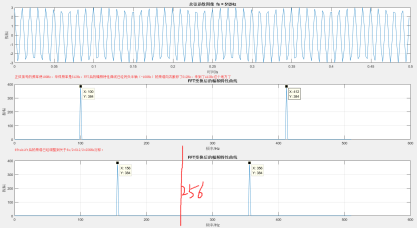对于x = 3*cos(2*pi*f1*t+thi1/180*pi) + 1*cos(2*pi*f2*t+thi2/180*pi);的幅频特性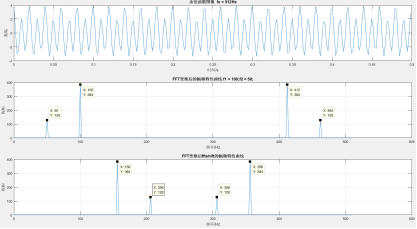对于x = 3*cos(2*pi*f1*t+thi1/180*pi) + 1*cos(2*pi*f2*t+thi2/180*pi);的相频特性与幅频特性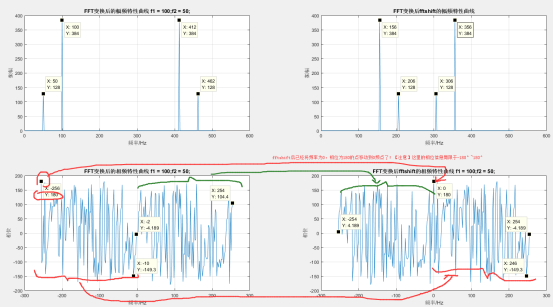Fftshift的作用：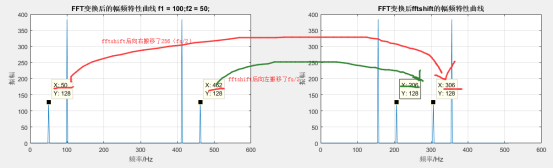结合图像来理解：http://blog.sina.com.cn/s/blog_5e1cdcaf0100yi41.html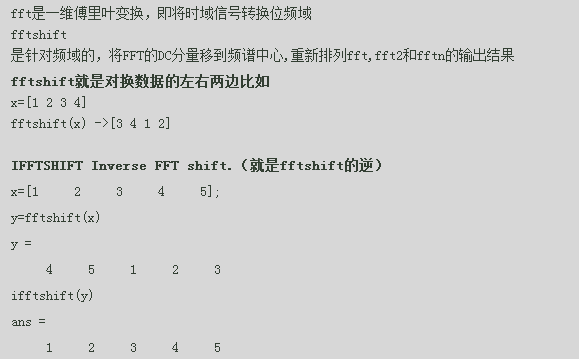2. 对于复数信号，且两个信号叠加的情况（要结合这份代码理解）：

%x=3*cos(2*pi*f1*t+thi1/180*pi)+1i*3*sin(2*pi*f1*t+thi1/180*pi)+1*cos(2*pi*f2*t+thi2/180*pi)+1i*1*sin(2*pi*f2*t+thi2/180*pi);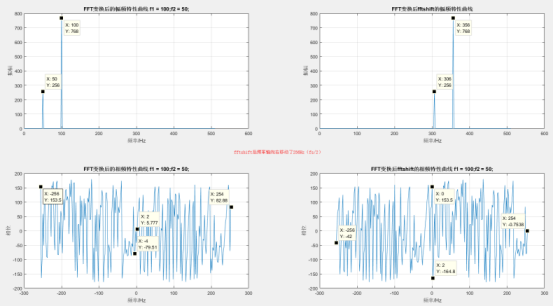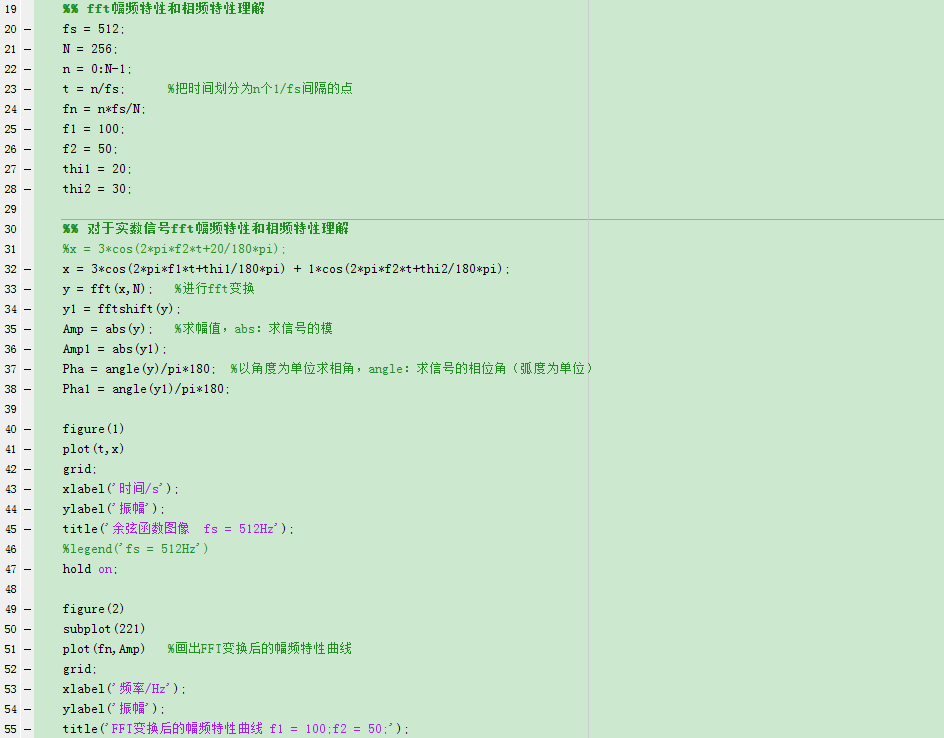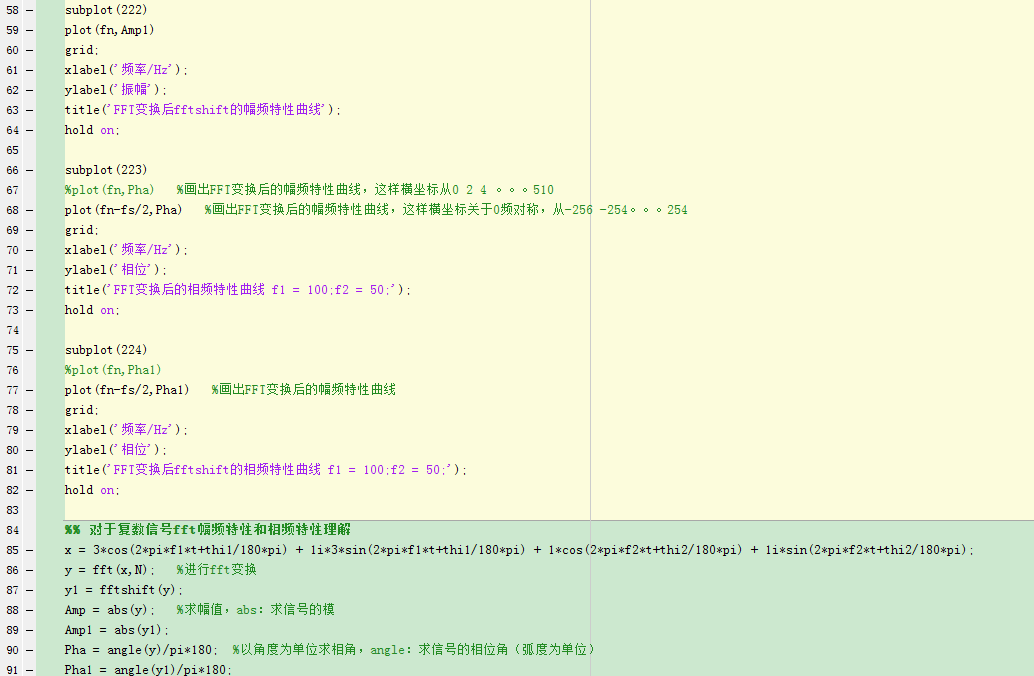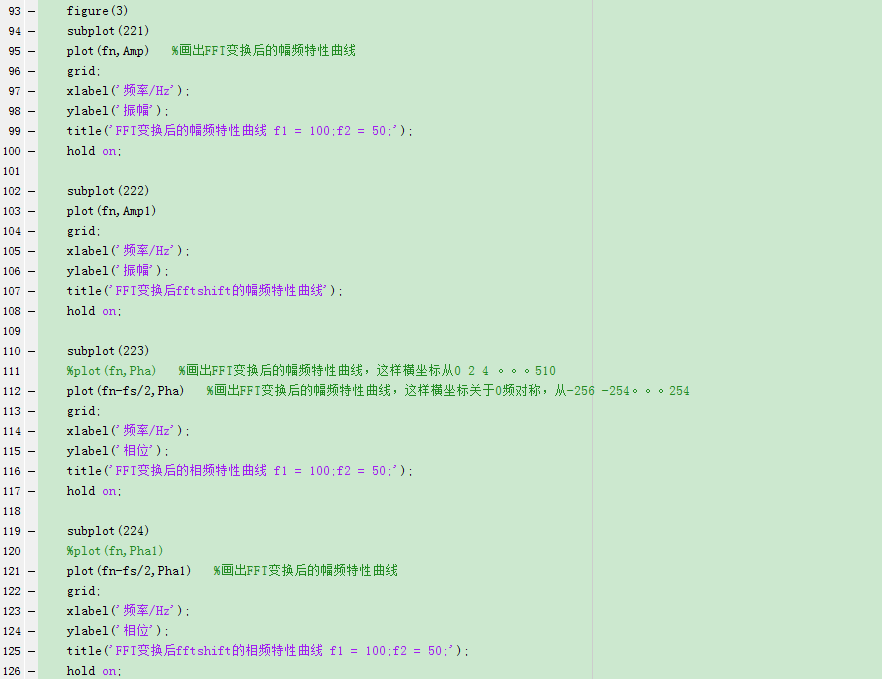相频响应的物理意义

研究一个线性时不变系统(LTI)，不仅可以用时域上的冲激响应h(n)来进行描述，也可以用频域上的频率响应H(w)来进行描述，而H(w)通常是一个复数，可分别用幅频响应和相频响应来表示。

幅频响应好理解，从物理概念上，幅频响应反映的是系统对不同频率信号的选择性。相频响应也有对应的非常明确的物理意义吗？回答是有的。从物理概念上，相频响应反映了系统对不同频率信号的处理时间。这点，我们也可以用一个例子来说明。

假定有甲乙丙三个同学，甲平时学习非常用功；乙平时除了学习，还喜欢关心很多其他的事情；丙则经常有点迷迷糊糊。三个人都报名参加了一个考试。到考试那天，甲乙都很早就起床，收拾妥当就往考点去了，丙则照常睡懒觉，等起来的时候都快到考试时间了，匆匆忙忙就往考点奔。这天考试的人很多，在进口处有保安查验证件，甲乙准备充分，很顺利地进入了考场，丙则因为太过匆忙，加上平时就经常犯迷糊，因为证件一时找不着了，连考场都没进得去。甲因为平时刻苦，很快就答完试卷出来了。乙则一直忙到考试结束。如果将考场当作一个系统，考生当作系统的输入。我们看到，甲乙两个信号都能通过系统，而丙则不能通过系统，这反映的是系统的选择性，即幅频响应。我们还看到，甲很快就出来了，乙则出来慢一些，这反映的是相频响应，即系统对不同信号的处理时间。

gy的：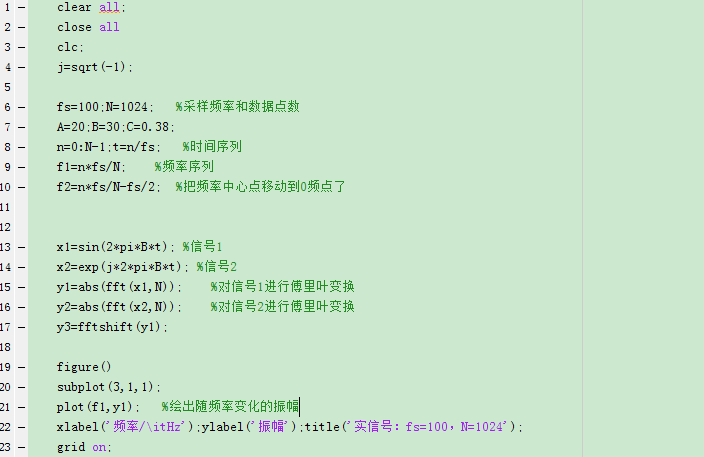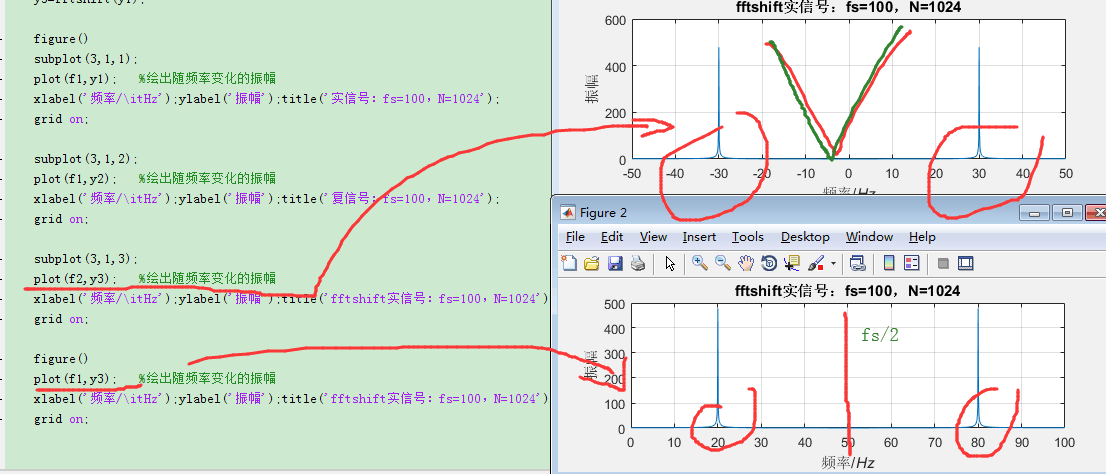上面第一幅图，实信号真实的频谱

展开全文• 基于STM32F407的幅频特性和相频特性测试仪，内含设计主要源码。
• 系统函数的幅频特性和相频特性分析参考与平台freqs(b,a,w)介绍例如输出 参考与平台 书籍《信号与系统》 matlab2016a freqs(b,a,w)介绍 对于有理分式，MATLAB 提供 freqs 函数处理方法。其调用格式为： H=freqs(b,a,w...
系统函数的幅频特性和相频特性分析参考与平台freqs(b,a,w)介绍例如输出
参考与平台
书籍《信号与系统》
matlab2016a
freqs(b,a,w)介绍
对于有理分式，MATLAB 提供 freqs 函数处理方法。其调用格式为：
H=freqs(b,a,w)
b 为分子多项式的系数，a 分母多项式的系数，w 为需计算的频率特性函数的取样点数。
例如
已知低通滤波器的频率特性函数为：
$H(jw)\;=\;\frac1{{(jw)}^3+2{(jw)}^2+2jw+1}$
求其幅频特性和相频特性。
w = linspace(0,5,200)
b = ;
a = [1 2 2 1];
H = freqs(b,a,w);
subplot(2,1,1);
plot(w,abs(H));
set(gca,'xtick',[0 1 2 3 4 5]);
set(gca,'ytick',[0 0.4 0.707 1]);
xlabel('\omega')
ylabel('|H(j\omega)|');
subplot(2,1,2);
plot(w,angle(H));
set(gca,'xtick',[0 1 2 3 4 5]);
xlabel('\omega')
ylabel('\phi(\omega)');

输出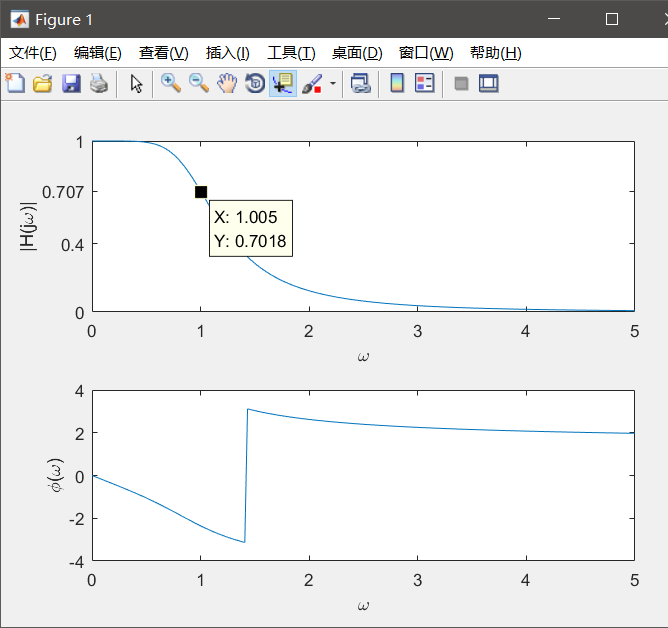展开全文• matlab画频率响应曲线的函数为： [h,w] = freqz(b,a,n) b,a：传递函数系数 h：频率响应 w：角频率，0~π ...用freqz画频率响应曲线的一个例子：绘制如下系统的频响曲线： H(z)=(1-0.5z^-1) ...Hf=a
matlab画频率响应曲线的函数为：

[h,w] = freqz(b,a,n)

b,a：传递函数系数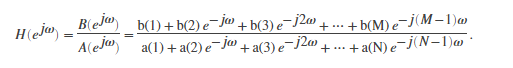h：频率响应

w：角频率，0~π

更多参数解释参考官方链接https://ww2.mathworks.cn/help/signal/ref/freqz.html?requesteddomain=true

用freqz画频率响应曲线的一个例子：绘制如下系统的频响曲线： H(z)=(1-0.5z^-1)

B=[1 -0.5];
A =;
[H,w]=freqz(B,A);
Hf=abs(H);  %取幅度值实部
Hx=angle(H);  %取相位值对应相位角
clf
figure(1)
plot(w,20*log10(Hf))  %幅值变换为分贝单位
title('离散系统幅频特性曲线')
figure(2)
plot(w,Hx)
title('离散系统相频特性曲线')

幅频特性曲线：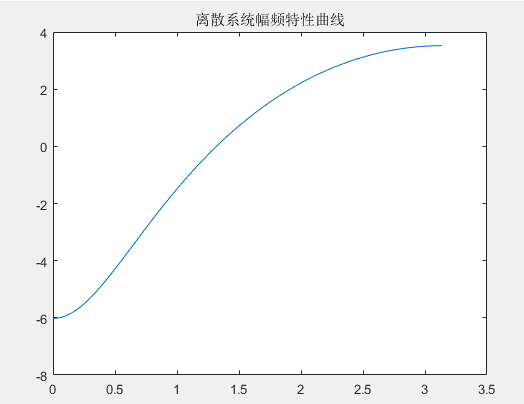此时幅频特性曲线的横坐标为数字角频率w。有时候我们想把横坐标转换为频率f（以赫兹hz为单位）：

首先要弄懂各个频率之间的关系：
模拟频率f：每秒经历多少个周期，单位Hz，即1/s；

各频率之间的关系：
Ω=2*π*f；

w =Ω*T；

因为T=1/fs（fs为采样率）

所以w =Ω*T=2*π*f/fs。

因此将数字角频率w转换为模拟频率f的公式为：

f=w*fs/(2*π)

因此，将横坐标转换为以赫兹为单位的代码如下（假设采样率为1000hz）：
B=[1 -0.5];
A =;
[H,w]=freqz(B,A);
Hf=abs(H);  %取幅度值实部
Hx=angle(H);  %取相位值对应相位角
clf
figure(1)
plot(w*fs/(2*pi),20*log10(Hf))  %幅值变换为分贝单位
title('离散系统幅频特性曲线')
figure(2)
plot(w*fs/(2*pi),Hx)
title('离散系统相频特性曲线')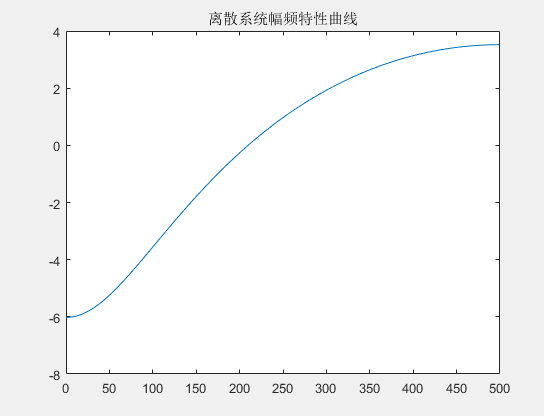此时幅频特性曲线的横坐标为频率f，单位为hz

ps：

当幅频特性曲线的横坐标为数字角频率w时，其最大值为π，这是因为当采样率为fs时，根据香农定理，能够采样的信号的最高频率为fs/2，fs/2频率对应的数字角频率w即为π。因此通常我们只关心0-π的幅频响应。

从上面最后一张图可以看到，当横坐标转换为频率后，横坐标最大值为fs/2，即500hz。

展开全文• 在反馈环路中增加一个主极点(参见图 7.9.2) ,并使它远离第二个极点,从而改变环路增益的频率特性,实现频率补偿。 例 7.8.1 设一电压放大电路的开环电压增益表达式为 A˙v=105(1+jf/105)(1+jf/106)(1+jf/107)\dot{A}_{...
**
康华光-《电子学技术基础-模拟部分》第367页
**
(1)增加主极点
在反馈环路中增加一个主极点(参见图 7.9.2) ,并使它远离第二个极点,从而改变环路增益的频率特性,实现频率补偿。
例 7.8.1
设一电压放大电路的开环电压增益表达式为
$\dot{A}_{v}=\frac{10^{5}}{\left(1+\mathrm{j} f / 10^{5}\right)\left(1+\mathrm{j} f / 10^{6}\right)\left(1+\mathrm{j} f / 10^{7}\right)}$
式中$10^5$是低频电压增益。 $\dot{A}_{v}$ 的频率响应波特图如图 7.8.4 所示,其主极点频率是$10^5$Hz 。将它组成负反馈放大电路,反馈网络由纯电阻组成,反馈系数$F_v=0.02$试分析该电路的工作稳定性,若不稳定,请用增加主极点的方法,
实现频率补偿。
书上的图是这样的，上面的转移函数对应下面波特图中的红线。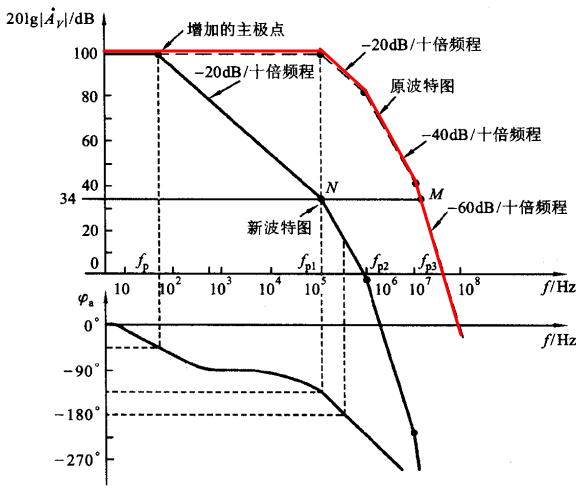我们尝试用matlab代码来实现
首先转化上述式子:
$jf=\frac{s}{2\pi}$
所以上述式子转化为:
$\dot{A}_{v}=\frac{10^{5}}{\left(1+\mathrm{j} f / 10^{5}\right)\left(1+\mathrm{j} f / 10^{6}\right)\left(1+\mathrm{j} f / 10^{7}\right)}$
三个极点:
$z_1=-2π*10^5$
$z_2=-2π*10^6$
$z_3=-2π*10^7$
所以
$\dot{A}_{v}=\frac{10^{23}(2π)^3}{(z+z_1)(z+z_2)(z+z_3)}$
Matlab代码如下:
z=[]'
p=[-2*pi*10^5 -2*pi*10^6 -2*pi*10^7]'
k=10^23*(2*pi)^3

[num,den]=zp2tf(z,p,k)
P=bodeoptions;
P.FreqUnits='Hz';

g=tf(num,den)
bode(g,P)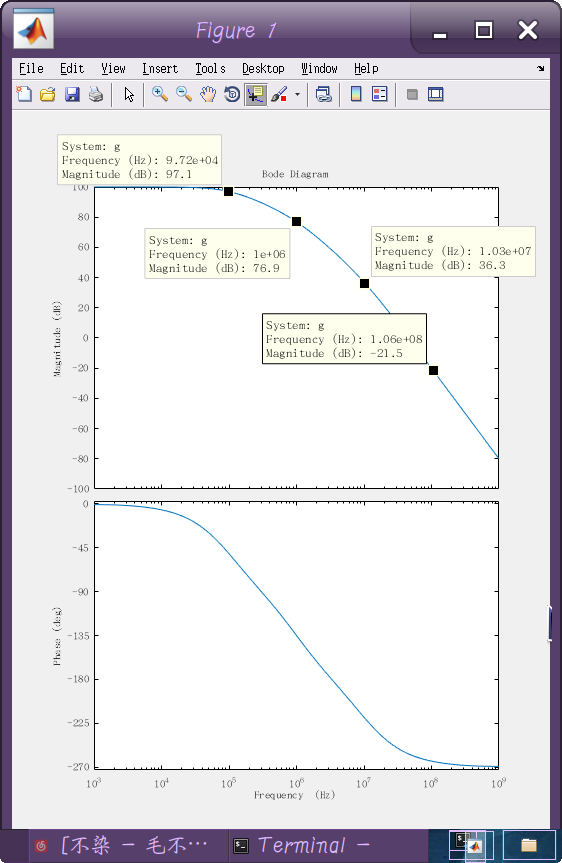根据上面读数可以看出，每经过一个极点，幅频下降斜率就会增加约
-20db/10倍频
因此可以验证书上的幅频图是正确的。
这里写matlab的时候需要注意两点:
①虽然代码中把频率单位调整为Hz，
但是输入的频率依然是角频率w，而不是$f$
②调整单位后，matlab已经自动帮你计算了$20log|\dot{A}_{v}|$
可以看到幅频图低通区域数值是100dB,与书上完全一致。


展开全文• 本程序使用matlab脚本分析闭环系统的频率特性，画出系统的幅频特性和相频特性曲线，即闭环伯德图。并直接得出该闭环系统的频率特性指标用来分析系统的稳定性。可以得到的指标有：谐振频率Wr、谐振峰值Mr、幅频带宽、...matlab
• 截止频率为14Hz的一阶RC二阶RC滤波器幅频相频特性及阶跃响应Matlab
• 信号幅频相频特性的画法(频率响应法)。频率响应法信号幅频相频特性的画法。信号与系统
• 频率特性测试仪是测量网络的幅频特性和相频特性，并显示相应曲线的一种快速、方便、动态、直观的测量仪器，可广泛应用于电子工程领域。 　该测试仪以扫频外差为基本原理，并以单片机和FPGA构成的最小系统为控制核心...
• 这里是题目: 低频频率特性测试仪 一、任务 设计并制作一个频率特性测试仪。 二、要求 1．基本要求 （1）制作一个幅频特性测试仪。其频率范围25kHz～40kHz；...计算网络的幅频和相频特性，用所制...
• 一个网络的频率特性包括幅频特性和相频特性，在系统设计时，各个网络的频率特性对该系统的稳定性、工作频带、传输特性等都具有重要影响。本设计采用数字频率合成技术产生扫频信号，以单片机和FPGA为控制核心，通过A...
• 　一个网络的频率特性包括幅频特性和相频特性，在系统设计时，各个网络的频率特性对该系统的稳定性、工作频带、传输特性等都具有重要影响。实际操作中，扫频仪大大简化了测量操作，提高了工作效率，达到了测量过程...
• 一个网络的频率特性包括幅频特性和相频特性，在系统设计时，各个网络的频率特性对该系统的稳定性、工作频带、传输特性等都具有重要影响。实际操作中，扫频仪大大简化了测量操作，提高了工作效率，达到了测量过程快速...FPGA
• 幅频特性和相频特性综合称为频率特性。测量频率的方法有点频法和扫频法。传统的模拟式扫频仪价格昂贵、体积庞大，不能直接得到相频特性，给使用带来诸多不便。为此，设计了数字扫频式频率特性测试仪。FPGA
• 信号的频谱是复数，包含幅频响应和相频响应，重复计算时的结果基本相同。 而随机信号的功率谱也可以对数据进行FFT，但必须计算模值的平方，因为功率谱是实数 图片来源 能量谱密度用于表示单位频...
• 采用DSP 和FPGA 架构的现代数字信号处理技术，设计了一台低成本，高度数字化和智能化的频率特性测试仪，实现了对20 Hz~150 MHz 范围内任意频段的被测网络幅频特性和相频特性测量和显示，完成了数据存储回放和传输，-...
• 仪器实现了对20Hz～150MHz范围内任意频段的被测网络幅频特性和相频特性测量、数据存储、回放、峰值查找以及-3dB测量，Q值查找等计算。由于大量采用大规模集成电路，不仅提高了系统的集成度，减小了体积，而目提升了...
• 基于直接数字频率合成技术的思想，采用现代...实现了对20Hz～150MHz范围内任意频段的被测网络幅频特性和相频特性测量。完成了数据存储、-3曲带宽计算、峰值查找等功能，幅度检测精度达到1dBm，相位检测精度1°等指标。
• 　在电子产品的生产和调试过程中，很多时候需要测量设备或网络的频率特性， 数字频率特性测试仪用于测量二端口线性非时变网络的频率特性， 包括幅频响应和相频响应。频率特性测量的关键是产生频率步进的扫频信号源，...
• 基于直接数字频率合成技术的思想，采用现代数字信号处理和显示技术，设计了一台低成本、数字化、智能化的频率特性测试仪。实现了对20Hz～150MHz范围内任意频段的被测网络幅频特性和相频特性测量。
• 本设计有源低通二阶滤波器(又称Sallen-Key 低通滤波器)...本文详细叙述了如何用计算法设计有源低通二阶滤波器的方法，并用仿真软件EWB进行电路仿真，得出了电路的幅频特性和相频特性，并进行了仿真分析和误差结果分析。
• 按频率响应G（jω）幅值，相位与频率的关系，分别给出幅频特性（用对数计算）和相频特性。 　幅频特性描述增益｜G（jω）｜与ω的函数关系：201g｜G（jω）｜-lgω。以分贝值（dB）为单位，其斜率用dB/dec表示（dec...
• R、C 组成的无源滤波器进行介绍，对各种无源RC 滤波器的幅频特性和相频特性进行分析。 然后对转折频率ωC =1000rad/s 的情形分别提出一个一阶RC 无源低、高通滤波器和一个二阶RC 无源低通滤波器的学生实验设计方案，...滤波
• 2、 熟悉线性相位FIR滤波器的幅频特性和相频特性； 3、 了解各种不同窗函数对滤波器性能的影响 4、 进行FIR、IIR滤波器的性能比较。 一、 三、实验要求 1、 调试实验程序，并且，给实验程序加注释； 2、 根据...matlab
• 2、 熟悉线性相位FIR滤波器的幅频特性和相频特性； 3、 了解各种不同窗函数对滤波器性能的影响 4、 进行FIR、IIR滤波器的性能比较。 一、 三、实验要求 1、 调试实验程序，并且，给实验程序加注释； 2、 根据...matlab
• 2、 熟悉线性相位FIR滤波器的幅频特性和相频特性； 3、 了解各种不同窗函数对滤波器性能的影响 4、 进行FIR、IIR滤波器的性能比较。 一、 三、实验要求 1、 调试实验程序，并且，给实验程序加注释； 2、 根据...matlab
• 2、 熟悉线性相位FIR滤波器的幅频特性和相频特性； 3、 了解各种不同窗函数对滤波器性能的影响 4、 进行FIR、IIR滤波器的性能比较。 一、 三、实验要求 1、 调试实验程序，并且，给实验程序加注释； 2、 根据...matlab...